﻿ Solving Fourth Order Boundary Value Problem by Using Extended Quantic B-spline InterpolationApplied Mathematics

p-ISSN: 2163-1409    e-ISSN: 2163-1425

2020;  10(2): 28-33

doi:10.5923/j.am.20201002.02

Received: Sep. 16, 2020; Accepted: Oct. 12, 2020; Published: Oct. 26, 2020### Solving Fourth Order Boundary Value Problem by Using Extended Quantic B-spline Interpolation

Intidhar Zamil Mushtt, Saad Shakir Mahmood, Dunya Mohamed Hamed

Mustansiriyah University, College of Education, Baghdad, Iraq

Correspondence to: Intidhar Zamil Mushtt, Mustansiriyah University, College of Education, Baghdad, Iraq.
 Email: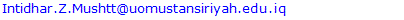Abstract

The aim of this paper is to solve the fourth order boundary value problem by using quantic b-spline where the rectangular system be solved by using singular value decomposition technique (SVD), and because the solution is not unique we used the least square optimization to optimal the numerical solution of the BVP. Numerical results are reported where we make a comparison between the exact solution and the approximate solution using the new technique.

Keywords: Quantic b-spline, Fourth order BVP, Approximate solution, Exact solution, SVD

Cite this paper: Intidhar Zamil Mushtt, Saad Shakir Mahmood, Dunya Mohamed Hamed, Solving Fourth Order Boundary Value Problem by Using Extended Quantic B-spline Interpolation, Applied Mathematics, Vol. 10 No. 2, 2020, pp. 28-33. doi: 10.5923/j.am.20201002.02.

1. Introduction
2. Conclusions

### 1. Introduction

Differential equations are an important tool in constructing mathematical models for physical phenomena. This modeling allows for a much clearer understanding and interpretation of the particular event. Finding the analytical and approximate solution of these models with boundary conditions thus becomes essential. Many analytical and approximate methods were developed solution of ordinary differential equation with boundary value conditions and a many these method as [1,2,3,4].
In this paper, the approximate solution of fourth order boundary value problem will be determine via Quantic B-spline, and comparisons with current studie will be made in the literature where the extended are given by the continuous least square optimization.
Since the fourth order B.V.P which is in the from: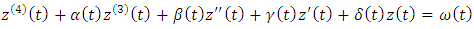(1)
Such that, and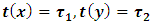Where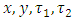are all constants, and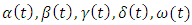are all a continuous functions defined on interval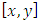.
Hence there has been much research activity concerning B-spline for solving boundary value problem we refer the reader [5,6,7,8,9,10].
Quantic B-spline interpolation method 
The interval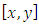of domain has been subdivided as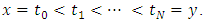To provide the support for the quantic B-spline near the end boundaries, ten additional knots have been introduced as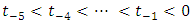and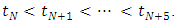The basis function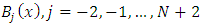of quantic B-spline are as: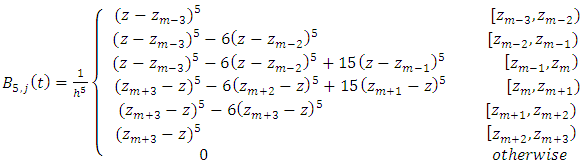(2)
The set of quantic B-spline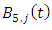form a basis over the region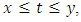the global approximation defined using quantic B-spline: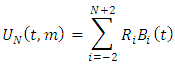(3)
By , The nodal value of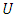and it's derivatives of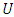to fourth order are given in terms of the parameters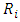from the use of spline (2) and the trial solution (3).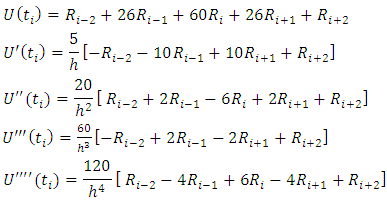Definition: 
Any real number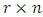matrix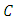can be decomposed as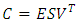where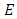is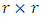and column orthogonal (it's column are eigenvectors of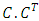)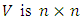and orthogonal (it's column are eigenvectors of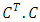)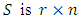diagonal (non-negative real values called singular values)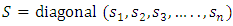order so that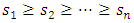(if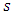is a singular value of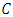it's square is an eigenvalue of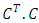).
Extended Quantic B-spline by using SVD and continuous least square error
Consider the Fourth order BVP: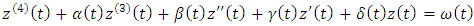On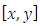such that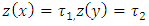, where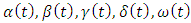are a continuous function defined on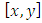, and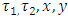are all constants.
The quantic B-spline is defined in equation (2)
Then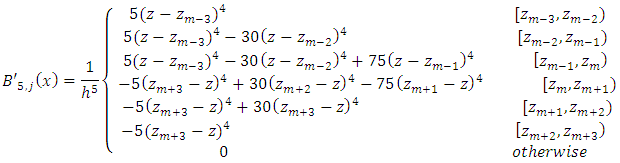And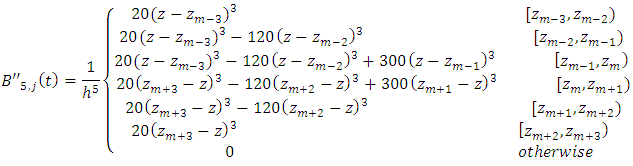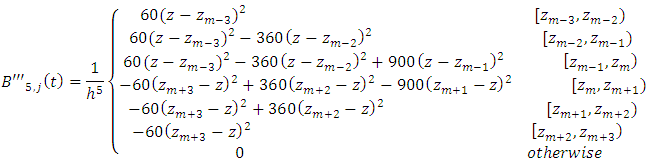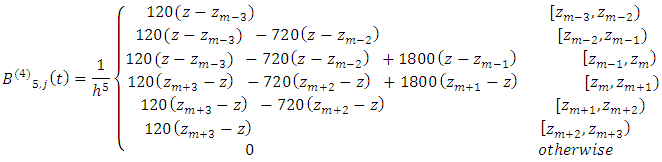Let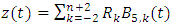, be an approximate solution of equation (1) where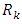is unknown real coefficients, let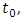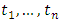are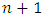grid points in the interval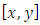and also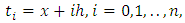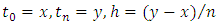in order to get a matrix of transactions that is contrary to the matrix of transaction that is contrary to the matrix from behind to make the image on the following form: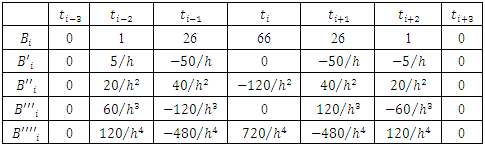This system can be written in matrix form as follows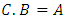such thatWhere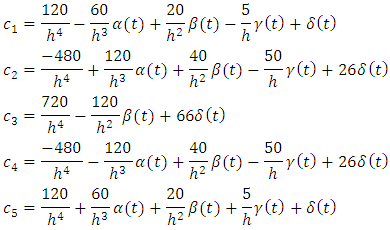And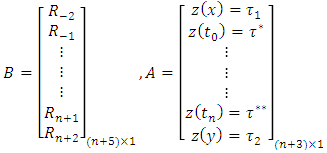The singular decomposition of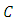has the form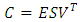so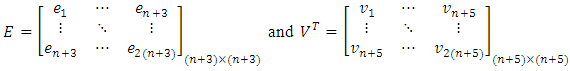and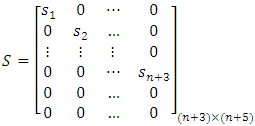So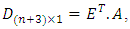let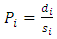then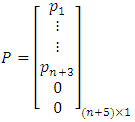and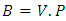Hence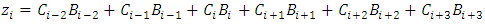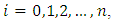then we have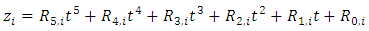be the solution of ODE by Quintic B-spline on the interval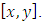The last equation can be solved by using continuous least square error to obtain the values of the constants with make the difference between the left-hand side and the right –hand side of BVP (1) is minimum.
Example 1: Consider the following ODE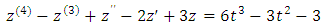With boundary conditions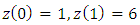and the exact solution is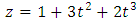Now let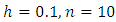then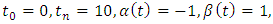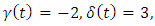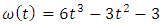This system can be written as follows:-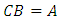Such that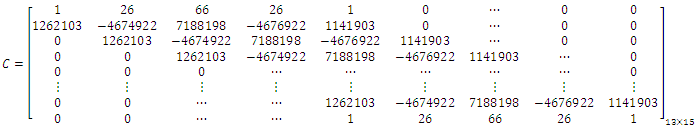And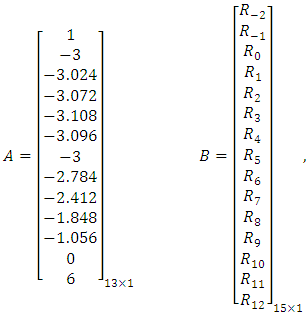Since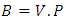be the values of the coefficient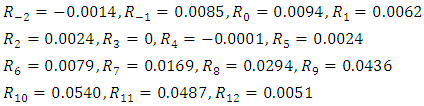Table (1). Shows the Extended B-spline with exact solution for the example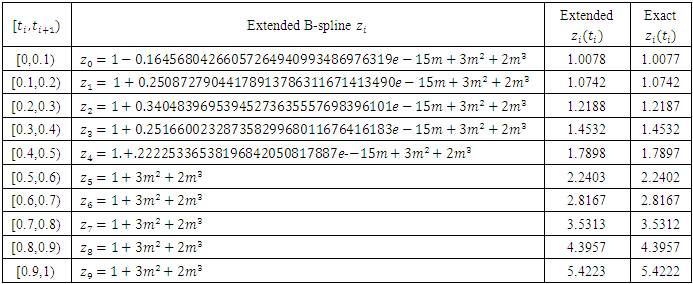### 2. Conclusions

Fourth order boundary value problem solved by using the extended quantic B-spline with continuous Least Square approximation and singular value decomposition technique. The numerical results showed that the extended quantic B-spline approximations are considered very well.

### References

  D. D. Demir and N. Bildik, the Numerical Solution of Heat Problem Using Cubic B-Splines, Apple. Math. 2012; 2(4): 131-135.  I. Dag, D. Irk and M. Sari, the Extended Cubic B-Spline Algorithm for Modified Regularized Long Wave Equation, chin. phys B. 2013; 22(4): 040207.  K. R. Raslan, T. S. El-Danaf and KH. K. Ali, Collection Method with Quintic B-Spline method for Solving the Hirota Equation, Jour of Abs. and Comp. Math. 2016; 1(1): 1-12.  O. Ersoy and I.Dag, Atrigonometric Cubic B-Spline Finite Element Method for Solving the Non Linear Coupled Burger Equation, Math. NA. 2016; 15: 1-18.  J. chang, Q. Yang,. Langzhao, Comparison of B-Spline Method and Finite Difference Method to Solve BVP of Linea ODEs, Jour of comp, 2011(6). 10: 2011.  K. N. Viswanadham and Y. S. Raju, Quintic B-Spline Collection Method for Sixth Order Boundary Value Problems, Glob. Jour., Inc. 2012; 12(1): 1-8.  F. G Lang, Quintic B-Spline Collection Method for Second Order Mixed Boundary Value Problem, Comp. phys. Com. 2012; 183(4): 913-921.  M. Munguia, D. Bhatta, Use of Cubic B-Spline in Approximating Solution of Boundary Value Problems, Apple. Apple. Math. 2015; 10(2): 750-771.  S. M. Reddy, Collection Method for Fifth Order Boundary Value Problem by Using Quintic B-Spline, Int. Jour of Eng. and Comp. Sci. 2016; 5 (8): 7696-17701.  R. K. Lodhi and H. K. Mishra, Quintic B-Spline Method for Solving Second Order Linear and nonlinear Singularly Perturbed Two Point Boundary Value Problems, Jour of Comp. and Apple. Math, 2017. 319. (1): 170-187.  S. S. Siddiqi and S. Arshed, Quintic B-Spline for the numerical Solution of Fourth-Order Parabolic Partial Differential Equation, World Apple. Sci. J. 2013. 23(12): 115-122.  I. Dag, B. Saka and A. Boz, Quintic B-Spline Galerkin Method for Numerical Solution of the Burgers' Equation, Dynamical system and applications proceeding. 2004: 295-309.  R. L. Burden and J. D. Fairs, Numerical Analysis, Youngstown State University, 2010.